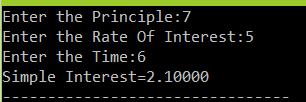This is the C program to calculate Simple Interest. We calculate simple interest to calculate interest charge on the loan. The following C program has been written and compiled on Dev C++.

#### Code:

#include<stdio.h>
void main(){
int t;
float p,r,si;
printf(“Enter the Principle:”);
scanf(“%f”,&p);
printf(“Enter the Rate Of Interest:”);
scanf(“%f”,&r);
printf(“Enter the Time:”);
scanf(“%d”,&t);
si=(p*r*t)/100;
printf(“Simple Interest=%.5f”,si);
}

OUTPUT:This site uses Akismet to reduce spam. Learn how your comment data is processed.